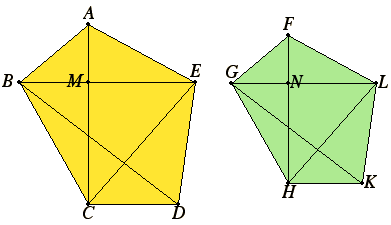# Proposition 20

Similar polygons are divided into similar triangles, and into triangles equal in multitude and in the same ratio as the wholes, and the polygon has to the polygon a ratio duplicate of that which the corresponding side has to the corresponding side.

Let ABCDE and FGHKL be similar polygons, and let AB correspond to FG.

I say that the polygons ABCDE and FGHKL are divided into similar triangles, and into triangles equal in multitude and in the same ratio as the wholes, and the polygon ABCDE has to the polygon FGHKL a ratio duplicate of that which AB has to FG.

Join BE, CE, GL, and HL.VI.Def.1

Now, since the polygon ABCDE is similar to the polygon FGHKL, therefore the angle BAE equals the angle GFL, and AB is to AE as GF is to FL.

Since then ABE and FGL are two triangles having one angle equal to one angle and the sides about the equal angles proportional, therefore the triangle ABE is equiangular with the triangle FGL, so that it is also similar, therefore the angle ABE equals the angle FGL.

But the whole angle ABC also equals the whole angle FGH because of the similarity of the polygons, therefore the remaining angle EBC equals the angle LGH.

And, since the triangles ABE and FGL are similar, BE is to AB as GL is to GF. Also, since the polygons are similar, AB is to BC as FG is to GH. Therefore, ex aequali, BE is to BC as GL is to GH, that is, the sides about the equal angles EBC and LGH are proportional. Therefore the triangle EBC is equiangular with the triangle LGH, so that the triangle EBC is also similar to the triangle LGH.

For the same reason the triangle ECD is also similar to the triangle LHK.

Therefore the similar polygons ABCDE and FGHKL have been divided into similar triangles, and into triangles equal in multitude.

I say that they are also in the same ratio as the wholes, that is, in such manner that the triangles are proportional, and ABE, EBC, and ECD are antecedents, while FGL, LGH, and LHK are their consequents, and that the polygon ABCDE has to the polygon FGHKL a ratio duplicate of that which the corresponding side has to the corresponding side, that is AB to FG.

Join AC and FH.

VI.6

Then since the polygons are similar, the angle ABC equals the angle FGH, and AB is to BC as FG is to GH, the triangle ABC is equiangular with the triangle FGH, therefore the angle BAC equals the angle GFH, and the angle BCA to the angle GHF.

I.32

And, since the angle BAM equals the angle GFN, and the angle ABM also equals the angle FGN, therefore the remaining angle AMB also equals the remaining angle FNG. Therefore the triangle ABM is equiangular with the triangle FGN.

Similarly we can prove that the triangle BMC is also equiangular with the triangle GNH.

V.22

Therefore, proportionally, AM is to MB as FN is to NG, and BM is to MC as GN is to NH. So that, in addition, ex aequali, AM is to MC as FN is to NH.

VI.1

But AM is to MC as the triangle ABM is to MBC, and as AME is to EMC, for they are to one another as their bases.

V.12

Therefore also one of the antecedents is to one of the consequents as are all the antecedents to all the consequents, therefore the triangle AMB is to BMC as ABE is to CBE.

V.11

But AMB is to BMC as AM is to MC, therefore AM is to MC as the triangle ABE is to the triangle EBC.

For the same reason also FN is to NH as the triangle FGL is to the triangle GLH.

And AM is to MC as FN is to NH, therefore the triangle ABE is to the triangle BEC as the triangle FGL is to the triangle GLH, and, alternately, the triangle ABE is to the triangle FGL as the triangle BEC is to the triangle GLH.

Similarly we can prove, if BD and GK are joined, that the triangle BEC is to the triangle LGH as the triangle ECD is to the triangle LHK.

V.12

And since the triangle ABE is to the triangle FGL as EBC is to LGH, and further as ECD is to LHK, therefore also one of the antecedents is to one of the consequents as the sum of the antecedents to the sum of the consequents. Therefore the triangle ABE is to the triangle FGL as the polygon ABCDE is to the polygon FGHKL.

VI.19

But the triangle ABE has to the triangle FGL a ratio duplicate of that which the corresponding side AB has to the corresponding side FG, for similar triangles are in the duplicate ratio of the corresponding sides.

V.11

Therefore the polygon ABCDE also has to the polygon FGHKL a ratio duplicate of that which the corresponding side AB has to the corresponding side FG.

Therefore, similar polygons are divided into similar triangles, and into triangles equal in multitude and in the same ratio as the wholes, and the polygon has to the polygon a ratio duplicate of that which the corresponding side has to the corresponding side.

Q.E.D.

# Corollary

Similarly also it can be proved in the case of quadrilaterals that they are in the duplicate ratio of the corresponding sides. And it was also proved in the case of triangles, therefore also, generally, similar rectilinear figures are to one another in the duplicate ratio of the corresponding sides.

## Guide

This proposition and its corollary are used occasionally in Books X, XII, and XIII, in particular, XII.1 and XII.8.